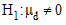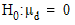# NMath User's Guide

41.4 Two Sample Paired T-Test (.NET, C#, CSharp, VB, Visual Basic, F#)

Class tests the null hypothesis that the population mean of the paired differences of two samples is zero. Pairing involves matching up individuals in two samples so as to minimize their dissimilarity except in the factor under study. Paired samples often occur in pre-test/post-test studies in which subjects are measured before and after an intervention. They also occur in matched-pairs (for example, matching on age and sex), cross-over trials, and sequential observational samples. Paired samples are also called matched samples and dependent samples.

NOTE—TwoSamplePairedTTest is equivalent to performing a OneSampleTTest on the paired differences (see Section 41.3).

For example, suppose we measure the thickness of plaque (mm) in the carotid artery of 10 randomly selected patients with mild atherosclerotic disease. Two measurements are taken: before treatment with Vitamin E (baseline), and after two years of taking Vitamin E daily. The mean difference between paired measurements is 0.045 with a standard deviation of 0.0264.

As described Section 41.1, all hypothesis test classes provide two paths for constructing instances of that type: a parameter-based method and a data-based method. Thus, you can construct a TwoSamplePairedTTest object by explicitly specifying the mean difference between paired observations (), the standard deviation of the differences (), and the sample size (), like so:

Code Example – C# paired t-test

```double xbar = 0.045;
```
```double s = 0.0264;
```
```int n = 10;
```
```var test = new TwoSamplePairedTTest( xbar, s, n );
```

Code Example – VB paired t-test

```Dim XBar As Double = 0.045
```
```Dim S As Double = 0.0264
```
```Dim N As Integer = 10
```
```Dim Test As New TwoSamplePairedTTest(XBar, S, N)
```

Alternatively, you can supply two sets of sample data. For instance, this code adds data to a DataFrame (Chapter 37):

Code Example – C# paired t-test

```var df = new DataFrame();
```
```df.AddColumn( new DFNumericColumn( "Baseline" ) );
```
```df.AddColumn( new DFNumericColumn( "Vit E" ) );
```
```df.AddRow( 1, 0.66, 0.60 );
```
```df.AddRow( 2, 0.72, 0.65 );
```
```df.AddRow( 3, 0.85, 0.79 );
```
```df.AddRow( 4, 0.62, 0.63 );
```
```df.AddRow( 5, 0.59, 0.54 );
```
```df.AddRow( 6, 0.63, 0.55 );
```
```df.AddRow( 7, 0.64, 0.62 );
```
```df.AddRow( 8, 0.70, 0.67 );
```
```df.AddRow( 9, 0.73, 0.68 );
```
```df.AddRow( 10, 0.68, 0.64 );
```

Code Example – VB paired t-test

```Dim DF As New DataFrame()
```
```DF.AddColumn(New DFNumericColumn("Baseline"))
```
```DF.AddColumn(New DFNumericColumn("Vit E"))
```
```DF.AddRow(1, 0.66, 0.6)
```
```DF.AddRow(2, 0.72, 0.65)
```
```DF.AddRow(3, 0.85, 0.79)
```
```DF.AddRow(4, 0.62, 0.63)
```
```DF.AddRow(5, 0.59, 0.54)
```
```DF.AddRow(6, 0.63, 0.55)
```
```DF.AddRow(7, 0.64, 0.62)
```
```DF.AddRow(8, 0.7, 0.67)
```
```DF.AddRow(9, 0.73, 0.68)
```
```DF.AddRow(10, 0.68, 0.64)
```

And this code constructs a TwoSamplePairedTTest from the two columns of data:

Code Example – C# paired t-test

```var test =
```
```   new TwoSamplePairedTTest( df[ "Baseline" ], df[ "Vit E" ] );
```

Code Example – VB paired t-test

```Dim Test As New TwoSamplePairedTTest(DF("Baseline"), DF("Vit E"))
```

The mean difference between paired measurements, the standard deviation, and the sample size are calculated from the given data.

In addition to the properties common to all hypothesis test objects (Section 41.1), a TwoSamplePairedTTest object provides the following read-only properties:

Xbar gets the mean of the differences between paired observations.

S gets the standard deviation of the differences between paired observations.

N gets the number of pairs.

DegreesOfFreedom gets the degrees of freedom.

By default, a TwoSamplePairedTTest object performs a two-sided hypothesis test () with. Non-default test parameters can be specified at the time of construction using constructor overloads, or after construction using the provided Type and Alpha properties.

Once you've constructed and configured a TwoSamplePairedTTest object, you can access the various test results using the provided properties, as described in Section 41.1:

Code Example – C# paired t-test

```Console.WriteLine( "t-statistic = " + test.Statistic );
```
```Console.WriteLine( "deg of freedom = " + test.DegreesOfFreedom );
```
```Console.WriteLine( "p-value = " + test.P );
```
```Console.WriteLine( "reject the null hypothesis? " + test.Reject);
```

Code Example – VB paired t-test

```Console.WriteLine("t-statistic = " & Test.Statistic)
```
```Console.WriteLine("deg of freedom = " & Test.DegreesOfFreedom)
```
```Console.WriteLine("p-value = " & Test.P)
```
```Console.WriteLine("reject the null hypothesis? " & Test.Reject)
```

The output is:

```t-statistic = 5.4
```
```deg of freedom = 9
```
```p-value = 0.000433006432003502
```
```reject the null hypothesis? True
```

This indicates that we can reject the null hypotheses (). We can conclude that the true mean thickness of plaque after two years treatment with Vitamin E is significantly different than before treatment.

Finally, remember that the ToString() method returns a formatted string representation of the complete test results:

```Two Sample t Test (Paired)
```
```--------------------------

```

```Mean of differences between pairs = 0.045
```
```Standard deviation of differences between pairs =
0.0263523138347365
```
```Sample size (number of pairs) = 10
```
```Computed t statistic: 5.4, df = 9

```

```Hypothesis type: two-sided
```
```Null hypothesis: true mean of differences between pairs = 0
```
```Alt hypothesis: true mean of differences between pairs != 0
```
```P-value: 0.000433006432003502
```
```REJECT the null hypothesis for alpha = 0.01
```
```0.99 confidence interval: 0.0179180371533991 0.0720819628466008
```

Top

Top